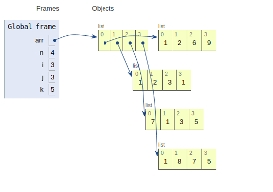# Python Program to Print Matrix in Z form

In this article, we will learn about the solution and approach to solve the given problem statement.

Problem statement −Given a square matrix of order n*n, we need to display elements of the matrix in Z form.

Z form is traversing the matrix in the following steps −

• Traverse the first row
• Now, traverse the second principal diagonal
• Finally, traverse the last row.

We will take an input matrix here implicitly taken to demonstrate the flow of code.demostrate

## Example

Live Demo

arr = [[1, 2, 6, 9],
[1, 2, 3, 1],
[7, 1, 3, 5],
[1, 8, 7, 5]]

n = len(arr)
i = 0
for j in range(0, n-1):
print(arr[i][j], end = ' ')
k = 1
for i in range(0, n):
for j in range(n, 0, -1):
if(j == n-k):
print(arr[i][j], end = ' ')
break;
k+= 1
# Print last row
i = n-1;
for j in range(0, n):
print(arr[i][j], end = ' ')

## Output

1 2 6 9 3 1 1 8 7 5

All variables and functions are declared in global scope as shown in the figure below.